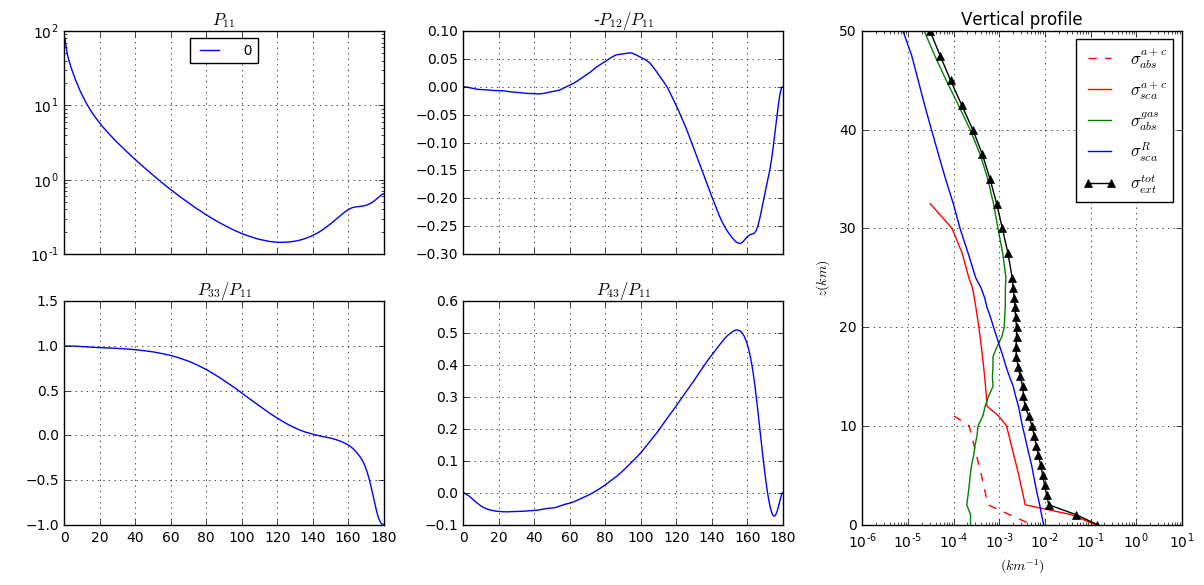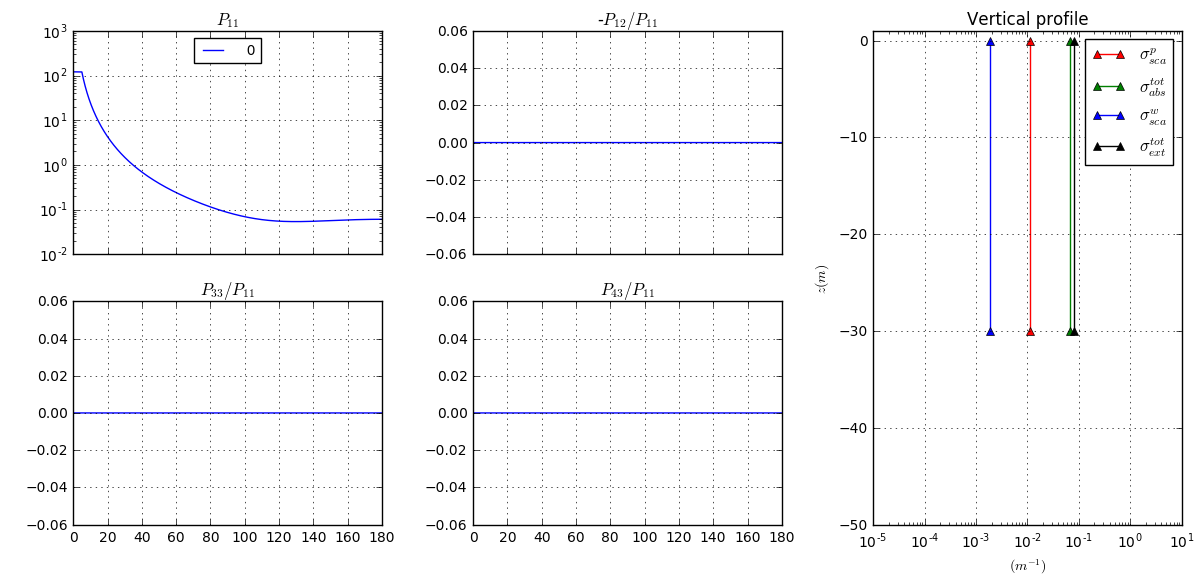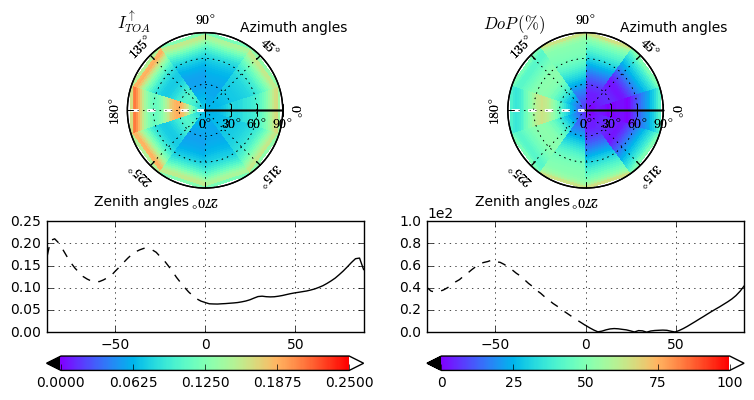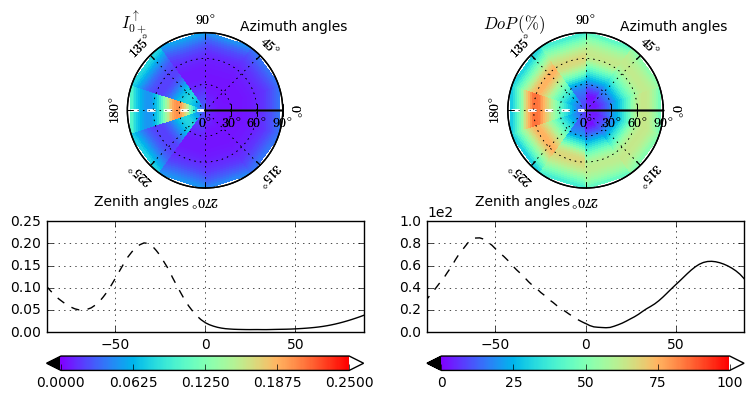# Simple Example¶

In [ ]:
# run SMART-G
# this simulation uses an AFGL tropical atmosphere without aerosols
# wavelength is 500 nm, number of photons is 10^9
# the surface is black
#
# Stokes vector computation in Cone Sampling mode (default)
m = Smartg(pp=True).run(   # use plane parallel geometry
THVDEG=45.,            # source zenith angle is 20°
wl=555.,               # wavelength is 555nm
NBPHOTONS=1e9,         # number of photons is 10^9
atm=AtmAFGL('afglt'),  # use an AFGL tropical atmosphere without aerosols
surf=None, water=None  # use a black surface
)

In :
fig=smartg_view(m, Imin=0.)
print ('kernel time for a GPU card Nvidia GTX 1070: %s (s)'%m.attrs['kernel time (s)'])

kernel time for a GPU card Nvidia GTX 1070: 3.67623406982 (s)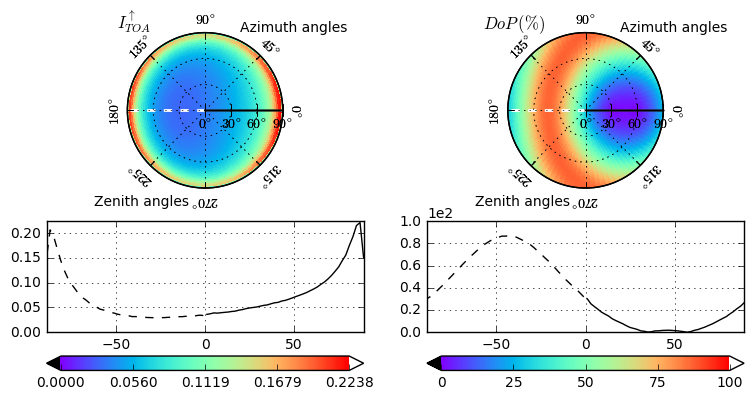In :
# this simulation uses an AFGL tropical atmosphere at a latitude of 0°, with aerosols, ozone (300 DU)
# and a surface pressure of 960 hPa.
# aerosols model "maritime polluted" from OPAC with an optical thickness of 0.2 at 555 nm

# the surface is a wind-roughened sea surface with a wind speed of 5 m/s,
# and the effect of wave shadows is included, index of refraction of water is 1.34.
# The ocean is considered homogeneous, typical of case 1 waters with a Chlorophyll a concentration
# of 0.1 mg/m3 and is 30 m deep. the sea bottom is a lambert reflector with a grey albedo of 0.2
# Stokes vector computation in Local Estimate mode
# the outputs directions are specified by the user : here Zenith angles (10) and Azimuth angles (40)
# equally spaced (so 400 directions)
# wavelength is 555 nm, number of photons is 10^6
# (in local estimate mode all photons contributes to the different directions,
# so less photons are necessary than for the Cone sampling mode)

S=Smartg()     # the Smartg object is created (compilation is done once for all)
wl_ref= 555.
wl= wl_ref
nvza=10
nraa=40
aer1 = AeroOPAC('maritime_polluted', 0.2, wl_ref)
atm  = AtmAFGL('afglms', lat=0., O3=300., P0=960., comp=[aer1])
water= IOP_1(chl=0.1, DEPTH=30., ALB=Albedo_cst(0.2))

le={}
le.update(phi_deg=np.linspace(0.,360., num=nvza, dtype=np.float32, endpoint=False))
le.update(th_deg= np.linspace(0.1,90., num=nraa, dtype=np.float32, endpoint=False))
m=S.run(THVDEG=30.,
wl=wl,
NBPHOTONS=1e6,
water=water,
atm=atm,
surf=surf,
le = le,            # activate Local Estimate mode
OUTPUT_LAYERS=3,    # compute also radiation fields at the surface (0+, 0-), sea bottom, upward and downward
progress=False)     # disable progress bar
print (m.attrs['kernel time (s)'])

22.3326367187

In :
# View input optical properties of the atmosphere and ocean
fig1=input_view(m,kind='atm')
fig2=input_view(m,kind='oc')

# View outputs polarized radiances for TOA and just above surface
fig3=smartg_view(m, Imax=.25, Imin=0., field='up (TOA)')
fig4=smartg_view(m, Imax=.25, Imin=0., field='up (0+)')